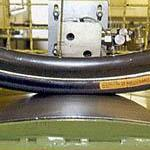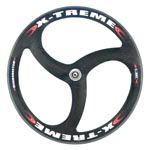Resistance of friction in cycling

For a cyclist, the friction (non-aerodynamic) Rm is the sum of two different resistances, the first comes from the contact of wheels on the ground and the second from mechanical pieces.

Contact of the wheels on the ground

The contact requires a sufficient grip to prevent falls but generates in return a rolling resistance Rr which is important. This resistance is proportionate to the total weight through a coefficient Cr and depends on the slope Pt (slope calculation). The total weight depends on the total mass Mt (cyclist plus equipment) and the acceleration of gravity g.

The coefficient defines the rolling efficiency of wheels. It depends on the nature of the ground (asphalt, cement, etc.) and the type of tires (constitution, form, size, inflation pressure, etc.).

 For standard wheels (700x23C), we can consider: Cr = 0.010 for a bad asphalt road Cr = 0.008 for a good asphalt road Cr = 0.006 for a very smooth asphalt road Cr = 0.004 for a velodrome trackFriction of mechanical pieces

The friction depends on the moving pieces, ie the wheels, crank, chain, sprocket, gears. Among all these things, the wheels generate the most important friction resistance Rf. It is is proportionate to the square of the speed relatively to the air Va through a coefficient Cf.

The coefficient defines the friction efficiency of wheels. It depends on the design (hub, spokes, rim). For example, there are wheels with profiled spokes, disc wheels.

 For standard wheels (700x23C), we can consider: Cf = 0.0030 for low-end wheels Cf = 0.0027 for good quality wheels Cf = 0.0024 for high-end wheelsN.B. The friction resistance is very low compared to others. The use of special wheels is justified only in extreme situations.

Calculation formula

In summary, the mathematic formula allowing calculation is:
Rm = Mt g Cr Cos(Arctan(Pt)) + Cf Va²

Calculation of the cycling power

J.L